#### You may also likeA 1 metre cube has one face on the ground and one face against a wall. A 4 metre ladder leans against the wall and just touches the cube. How high is the top of the ladder above the ground?In this problem we are faced with an apparently easy area problem, but it has gone horribly wrong! What happened?### From All Corners

Straight lines are drawn from each corner of a square to the mid points of the opposite sides. Express the area of the octagon that is formed at the centre as a fraction of the area of the square.

# Which Is Cheaper?

##### Age 14 to 16Challenge Level

Which car park should I use?
Aida, Mark and Adrien from Kings College Alicante in Spain, Anh Minh from British Vietnamese International School in Vietnam and Muhammad from Dubai International Academy in UAE compared car parks A and B. Anh Minh sent these two tables: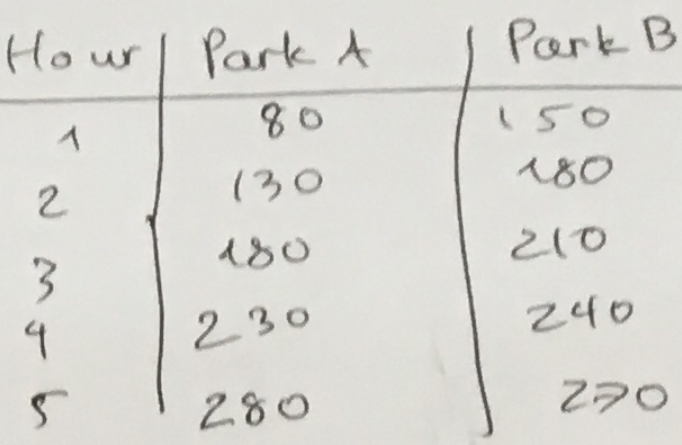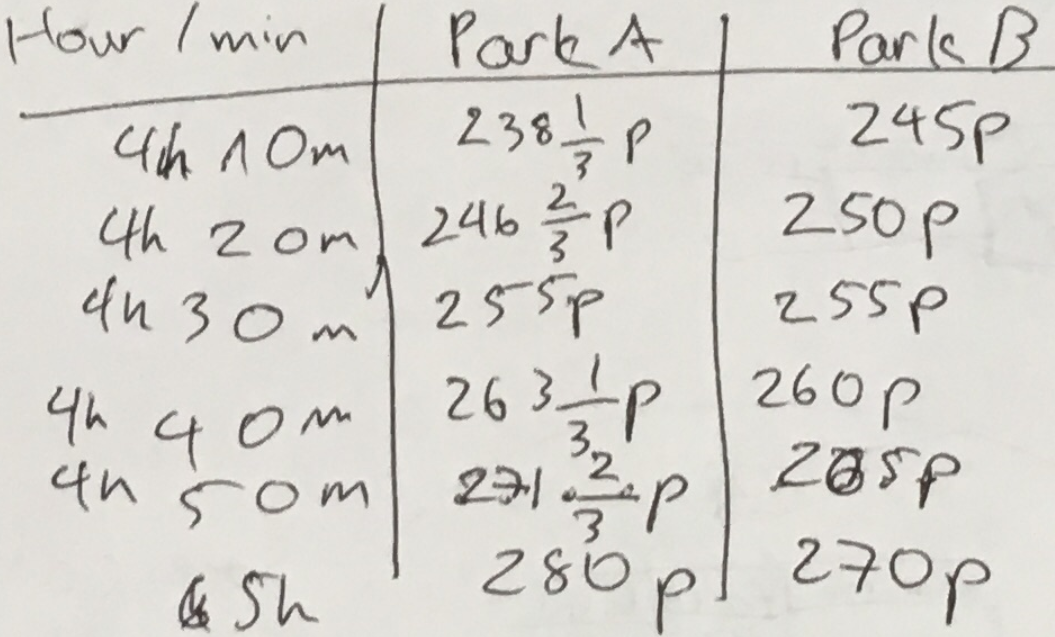Conclusion: if you park for more than 4 h 30 min, choose car park B, if less that 4 h 30 min, choose park A.

Adithya from Hymers College in the UK and Connor from Tanglin Trust School in Singapore used tables to compare all four options. Here is Connor's table: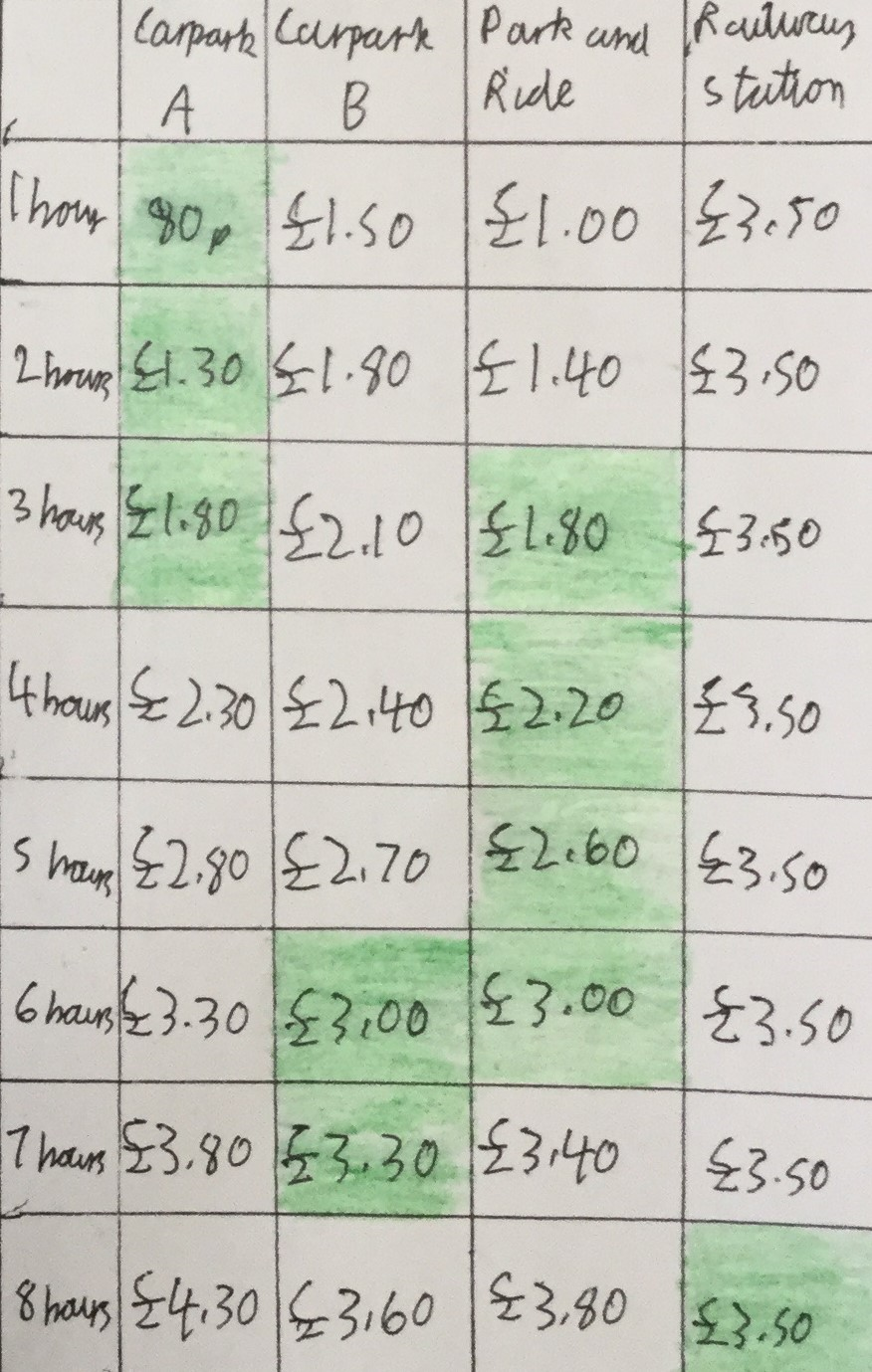If you are staying for 1 - 3 hours, car park A is cheaper. If you are staying for 3 - 6 hours, park and ride is cheaper. If you are staying for 6 - 7 hours, car park B is cheaper. If you are staying for more than 8 hours, taking the train is cheaper.

Anh Pham from British Vietnamese International School in Vietnam used algebra and inequalities to find which car park was cheapest:
Assume that the hours of parking car is: $n$ (hours, $n$ is an integer because the cost is calculated each hour)
1 pound     = 100p
At the Car park A:
The cost is: $A=80+50(n-1)$
$=30+50n$
At the Car park B:
The cost is: $B=150+30(n-1)$
$=120+30n$
At the Park and Ride:
The cost is: $P=60+40n$
At the train station:
The cost is: $T=350$
1. A is smallest
\begin{align}A&\lt B\\ 30+50n&\lt120+30n\\ 20n &\lt 90\\ n &\lt 4.5\end{align}

\begin{align}A &\lt P\\ 30+50n&\lt60+40n\\ 10n &\lt 30\\ &\lt 3 \end{align}

\begin{align}A&\lt T\\ 30+50n&\lt350\\ 50n &\lt 200\\ n &\lt 4\end{align}

$\Rightarrow$ A is smallest when $n\lt 3$

2. B is smallest
$B\lt A$ when $4.5\lt n$ (from before)

\begin{align}B&\lt P\\ 120+30n&\lt60+40n\\ 60 &\lt 10n\\ 6 &\lt n\end{align}

\begin{align}B&\lt T\\ 120+30n&\lt350\\ 30n &\lt 230\\ n &\lt \frac{23}{30}=7.7\end{align}

$\Rightarrow$ B is smallest when $6\lt n\lt7.7$
$\Rightarrow n=7$ ($n$ is an integer)

3. P is smallest
$P\lt A$ when $3 \lt n$ (from before)

$P\lt B$ when $n \lt 6$ (from before)

\begin{align} P&\lt T\\ 60+40n&\lt350\\ 40n &\lt 290\\ n &\lt \frac{29}4=7.25\end{align}

$\Rightarrow$ P is smallest when $3\lt n\lt 6$

4. T is smallest
$T\lt A$ when $6.4\lt n$
$T\lt B$ when $7.7\lt n$
$T\lt P$ when $7.25\lt n$
$\Rightarrow$ T is smallest when $n\gt7$

5. If $n=6$
$\Rightarrow B=P=300\lt A\lt T$

Result:
If you park for less than 3 hours, Car park A is the cheapest
If you park for more than 3 hours but less than 6 hours, Park and Ride is the cheapest
If your park for exactly 6 hours, Car park B and Park and Ride are the cheapest
If your park for exactly 7 hours, Car park B is the cheapest
If your park for more than 7 hours, Train station is the cheapest

Siddhant from Singapore International School Mumbai in India and Leopold from Kings Al Barsha in UAE made small mistakes, but went on to do good work. Siddhant used the following equations:
Car Park A:
In car park A, it costs 80p to park for the first hour, and an extra 50p
for each hour after that.
Therefore 0.80 is the fixed amount and 0.50 is the amount that is dependant
on the number of hours, or $x$. The expression you get is:$$0.80 + 0.50x$$
Car Park B:
In car park B, it costs £1.50 to park for the first hour, and an extra 30p
for each hour after that.
Therefore 1.50 is the fixed amount and 0.30 is the amount that is dependant
on the number of hours, or x. The expression you get is: $$1.50 + 0.30x$$
Park and Ride service:
In the Park and Ride service, it costs 40p per hour to park, and 60p for
the bus fare into town. Since there are two trips, the fixed amount wil be
1.20, and the amount dependant on the number of hours is 0.40. The
expression you get is: $$1.20 + 0.40x$$
For car parks A and B, Siddhant has used $x$ to represent the number of hours after the first hour. For park and ride, Siddhant has used $x$ to represent the total number of hours. Leopold made the same mistake, but assumed that you paid 60p for the total bus fare, not 60p each way (there is no right or wrong interpretation of the bus fare).

Siddhant and Leopold both went on to produce graphical solutions, which contain the same mistake, but are still useful representations. This is Siddhant's graph: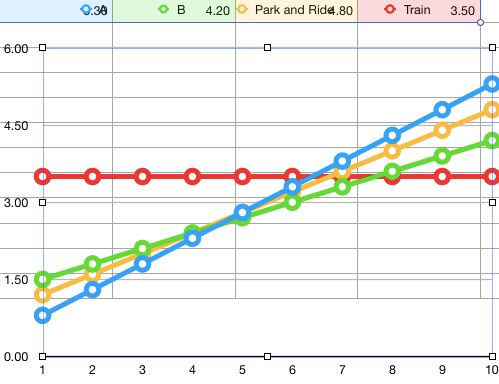Here is a corrected graph (with the same colours - car par A: blue, car park B: green, park and ride: orange, train: red):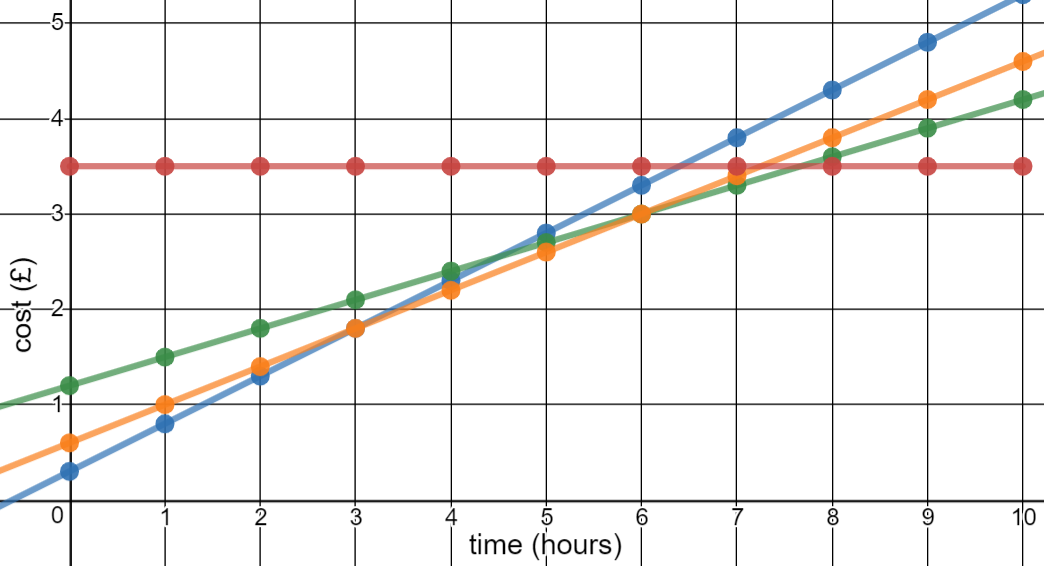Gower, Ata, Tom, Morgan, Jonathan and Mitra from Ashmole Academy all got the same answers as Siddhant, and Callum and Cooper from Wantirna College in Australia and Aida, Mark and Adrien got the same answers as Leopold.

Jathusha from Harris Girls' Academy Bromley in the UK included some real life aspects of the problem:

There are three options Car Park A (is cheaper compared to Car Park B), Train and the Park and Ride. If I were to choose I would go for Car Park A because the Train is too costly and Park and Ride is cheap BUT when I leave the car and then take a bus then the bus will take a while to come and I will have to pay for the car park more because of waiting for the bus twice (to and from) and how long I stay at Mathstown. Therefore, I would choose Car Park A so that I can travel to the centre of Mathstown and visit how long I need to and pay the expenses.
Nobody else had remembered that you still have to pay for the time your car is in the park and ride car park, but you have not yet reached the town centre!

Adithya had an idea for the charging structures of car parks I, J and K: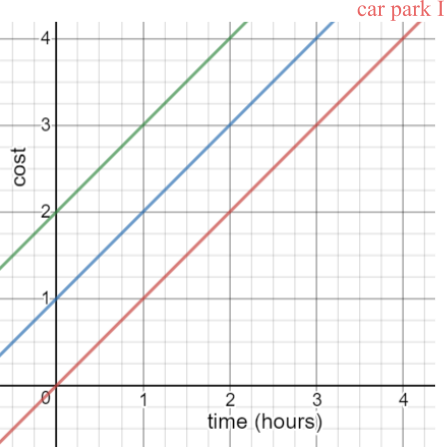Graphically, all these lines would be parallel and therefore the cheapest one is the one with the lowest $y$ intercept. Thus, the charging structures of these three car parks could come in the form of $x$, $x+1$ and $x+2$ and any corresponding multiples of these three expressions.

For example, car park I costs £1 per hour, car park J costs £2 for the first hour and then £1 per hour, car park K costs £3 for the first hour and then £1 per hour.

For the other car parks described in the challenge, Aida, Mark and Adrien sent us their method for finding charging systems:

A graph can be created which represents the price per hour.
Symbols used to represent the hourly increase in cost (representing gradients): $\mu, \eta, \kappa$
Symbols used to represent the initial cost of parking (representing intercepts): $c, z, g$
1) Create expression for total price, with the terms of gradient, intercept, and $n$
($= h-1$) (where $h$ represents the number of hours spent at the car park).
2) Create an equation for the time when the total prices for two parking are equal.
3) We can find out that time period by looking at which hour a car park stops being
cheaper than another, by finding the time period at which their prices are equal.
4) Substitute $n$ in the equation by that value minus one (as $n = h-1$).
5) As you have three/four unknowns, there is no 'one and only' solution, but you can find one which fits.
6) Replace one of the gradients by any reasonable value ($£0.10$ - $0.90$). Choose a suitable value for the other gradient, making sure it is lower or higher than the first
one, as specified.
7) Try to find values for the intercepts $c$ and $z$ that are integers and fit in to make the equation work (both sides should be equal).
8) If there is another equation afterwards, repeat the same process. However, as you have already chosen the value for the gradient and intercept of one, substitute these value into the equation instead of finding new ones.
9) Choose values for the gradient and intercept of the other sum.

Two car parks A and B, such that car park A is cheaper if you park for less than 5 hours and car park B is cheaper if you park for more than 5 hours.
$A = n(\mu) + c$  ($\mu\gt \eta$)
$B = n(\eta) + z$
$A$ and $B$ have to be equal at $h = 5$  ($n=4$)
$4\mu+c = 4\eta+z$
eg:
Let $\mu = 0.40, c = 0.10$
And $\eta = 0.30, z = 0.50$
$A = 0.40n +0.10$
$B = 0.30n +0.50$
Car Park A charges $£0.10$ the first hour and an additional $£0.40$ for each subsequent hour.
Car Park B charges $£0.50$ the first hour and an additional $£0.30$ for each subsequent hour.

Three car parks C, D and E, such that car park C is cheapest if you park for less than 2 hours, car park D is cheapest if you park for between 2 and 6 hours, and car park E is cheapest if you park for more than 6 hours.
$C = n(\mu)+c$  ($\mu\gt \eta\gt \kappa$)
$D = n(\eta)+z$E = n(\kappa)+g
$D$ and $C$ have to be equal at $h = 2$  ($n=1$)
$\mu+c = \eta+z$
eg:
Let $\mu = 0.50, c = 0.20$
And $\eta = 0.40, z = 0.30$
$D$ and $E$ have to be equal at $h = 6$  ($n=5$)
$5\eta+z = 5\kappa+g$
As $Å‹ = 0.40, z = 0.30$
Let $Ä¸ = 0.30, z = 0.80$
$C = 0.50n +0.20$
$D =0.40n +0.30$
$E = 0.30n+0.80$
Car Park C charges $£0.20$ the first hour and an additional $£0.50$ for each subsequent hour.
Car Park D charges $£0.30$ the first hour and an additional $£0.40$ for each subsequent
hour.
Car Park E charges $£0.80$ the first hour and an additional $£0.30$ for each subsequent hour.

Three car parks F, G and H, such that car park F is cheapest if you park for less than 3 hours, car park G is the cheapest if you park for more than 3 hours, and car park H is never cheapest.
$F = n(\mu)+c$  ($\kappa\gt \mu \gt \eta$), ($\kappa+g \gt \mu+c$), ($\kappa+g \gt \eta+z$) (the two new inequalities are to ensure car park H is always more expensive than both the other car parks; in fact, you need $g \gt c$ and $g \gt z$ because $n=0$ when $h=1.$
$G = n(\eta)+z$
$H = n(\kappa)+g$
$F$ and $G$ have to be equal at $h = 3$  ($n=2$)
$2\mu+c = 2\eta+z$
eg:
Let $\mu = 0.40, c = 0.10$
And $\eta = 0.35, z = 0.20$
Let $\kappa = 0.50, g = 0.40$
$F = 0.40n +0.10$
$G = 0.35n+0.20$
$H = 0.50n +0.40$
Car Park F charges $£0.10$ the first hour and an additional $£0.40$ for each subsequent hour.
Car Park G charges $£0.20$ the first hour and an additional $£0.35$ for each subsequent hour.
Car Park H charges $£0.40$ the first hour and an additional $£0.50$ for each subsequent hour.

Three car parks I, J and K, such that car park I is always cheaper than car parks J and
K, regardless of how long you park.

$I = n(\mu)+c$  ($\mu\lt \eta$),($\mu\lt \kappa$),($\mu+c \lt \eta+z$),($\mu+c \lt \kappa+g$)
$J = n(\eta)+z$
$K = n(\kappa)+g$
The initial price for one hour for car park I has to be lower than the price for one hour for the other two parks, and the additional cost for each hour also has to be lower for I than for J and K.
eg:
$I = 0.15n+0.10$
$J = 0.30n+0.25$
$K = 0.25n+0.20$
Car Park I charges $£0.10$ the first hour and an additional $£0.15$ for each subsequent hour.
Car Park J charges $£0.25$ the first hour and an additional $£0.30$ for each subsequent hour.
Car Park K charges $£0.20$ the first hour and an additional $£0.25$ for each subsequent hour.

We received some other suggestions for car parks C to H which had very unusual charging systems. Sometimes, you had to pay less if you stayed for longer, and sometimes the car parks paid customers to leave their cars there!